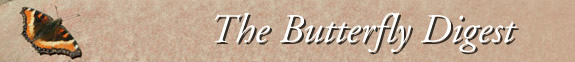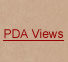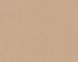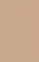Jump to : Today May 17, 2022 May 16, 2022 May 15, 2022 May 14, 2022 May 13, 2022 May 12, 2022 May 11, 2022 May 10, 2022 May 9, 2022 May 8, 2022 May 7, 2022 May 6, 2022 May 5, 2022 May 4, 2022 May 3, 2022 May 2, 2022 May 1, 2022 April 30, 2022 April 29, 2022 April 28, 2022 April 27, 2022 April 26, 2022 April 25, 2022 April 24, 2022 April 23, 2022 April 22, 2022 April 21, 2022 April 20, 2022 April 19, 2022 April 18, 2022     |    View All Lists    |    FAQ
Tha=nks=20Harry Pavulaan for this timely and important report. It is= much appreciated!

Jeremy Coleman
Shelburne= Falls

On Sat, Apr 23, 2022 at 12:37 PM pavulaan via MassLep &l=t;">&=gt; wrote:
Spring greetings:

I=E2=80=99m pleased to announce publication of The Taxonomic Report Vo=l. 10, No. 2.=C2=A0 This is a TTR =E2=80=98Notes on Eastern North American =Lepidoptera=E2=80=99 issue with three reports.=C2=A0 I am forwarding this t=o Masslep because I've seen more frequent images of what appear to be s=pring form Summer Azures appearing in New England.=C2=A0 Hopefully, the att=ached paper will help folks distinguish Summer Azures flying among Spring A=zures.=C2=A0 In many areas of the Virginia and Maryland Piedmont, Spring Az=ures (C. ladon) have disappeared d=ue to demise of their host, Flowering Dogwood, due to Dogwood Anthracnose.==C2=A0 The Summer Azure (C. neglecta) has developed a full spring brood and has essentially become our "=Spring Azure", thus replacing the original Spring Azure in its ecologi=cal niche.
=C2=A0
=20
1. =E2=80=98Celastrina ladon andC. neglecta are distinct species differentiated primarily by a diagn=ostic wing scale structure, voltinism, and host tolerance ofCornus florida=E2=80=99 by Harry Pavulaan.
=C2=A0
=20
While most people in the realm of butterfly study/observation now reco=gnize the Spring (C. ladon) and Summer (C. neglecta) Azures a=s distinct species, there is still confusion over how to distinguish them f=rom each other.=C2=A0 There are also =E2=80=9Choldouts=E2=80=9D that, despite several papers on =the topic and most field guides now separating these as full species =E2=80==93 still insist that the differences are merely individual variation and n=eed more =E2=80=9Cproof=E2=80=9D to understand the clear differences.=C2=A0 This paper attempts, in the clearest terms, to present these obvious diffe=rences.=C2=A0 An especial thanks to members of MDLepsOdes who provided a gr=eat many =E2=80=9CSpring=E2=80=9D Azure images (most of which happened to b=e Summer Azures).=C2=A0 It was difficult deciding which =E2=80=9Clive=E2=80==9D images to use in the paper.

One word of caution for Massachusetts butterfliers:=C2=A0 Spring Azure=s (C. ladon) take on the appearanc=e of C. lucia (Northern Spring Azu=re) in southern New England.=C2=A0 They frequently develop both dark patche=s and dark margins on the underside of the hindwings, as well as just showi=ng the spotted form (which is illustrated in the attached paper).=C2=A0 How=ever, it is the male forewing upperside that distinguishes the Spring Azure=.=C2=A0 When one encounters a spring form Summer Azure (C. neglecta), these are characterized by their very w=hite underside and reduced spot pattern.=C2=A0 They will fly among the Spri=ng Azures.=C2=A0 Not to be confused with the Cherry Gall Azure (C. serotina), that appears similar to both Sp=ring and Summer Azures, but emerges later - in May.=C2=A0=C2=A0

DNA work has been underway on all North American Azures and will be pu=blished sometime soon.=C2=A0 Regardless of similar appearances, the study s=upports separating these as full species.
=C2=A0
=20
1. =E2=80=98A rare case of mosaic gynandromorphism in the Zabulon Skipper =(Lon zabulon)=E2=80=99 by Annette Allor.
=C2=A0
=20
This was a fascinating discovery last year by Annette Allor.=C2=A0 It =is a rare case of mixed male/female features in a Skipper butterfly, and ma=y be the only published case of this happening in North America among the S=kippers, though there is one case of bilateral gynandromorphism that I am aware of.=C2=A0 This r=eport also highlights the value of photographic documentation of natural hi=story experiences.=C2=A0 My thanks to Annette for bringing this to my atten=tion!
=C2=A0
=20
1. =E2=80=98Rhode Island, USA Fall Lepidoptera Survey 2021=E2=80=99 by Har=ry Pavulaan (coordinator)=E2=80=99.
=C2=A0
=20
While butterfly counts abound, mainly around July 4th, an a=ttempt was made to document lepidoptera activity in the Fall of 2021, in Rh=ode Island.=C2=A0 The survey period focused on Sept. 18-Oct. 10, a time dur=ing which lepidopteran migratory activity is at a peak, and fall broods are in full flight.=C2=A0= A complete list from several direct participants is compiled, and addition=al reports from iNaturalist and the R.I. Butterflies and Moths Facebook gro=up are included.
=C2=A0
=20
Thanks to all who have provided support to TILS!=C2=A0
=C2=A0
=20
Harry Pavulaan
=C2=A0
=20
Sent from Mail for Windows
=C2=A0
=20

--
You received this message because you are subscribed to the Google Groups &=quot;MassLep" group.
To unsubscribe from this group and stop receiving emails from it, send an e=mail to " target=3D"_=blank">masslep+.
To view this discussion on the web visit ?utm_medi=um=3Demail&utm_source=3Dfooter" target=3D"_blank">https://groups.google=.com/d/msgid/masslep/<424788534.347689.1650731858666...>.

--
You received this message because you are subscribed to the Google Groups &=quot;MassLep" group.
To unsubscribe from this group and stop receiving emails from it, send an e=mail to ">masslep+uns=.
To view this discussion on the web visit ?utm_medium=3Demail&utm_source=3Dfooter">https://groups.g=oogle.com/d/msgid/masslep/CAOn0--mc%3DMEavo-oz71VHN08VKN5%2BNAu9zhg19%2BJ4V=.
--0000000000007d0f8405dd58374c--